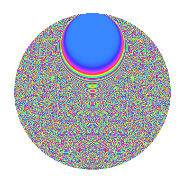# Properties

 Label 3024.2.gvLevel 3024 Weight 2 Character orbit gv Rep. character $$\chi_{3024}(293,\cdot)$$ Character field $$\Q(\zeta_{36})$$ Dimension 6864 Sturm bound 1152

# Related objects

## Defining parameters

 Level: $$N$$ = $$3024 = 2^{4} \cdot 3^{3} \cdot 7$$ Weight: $$k$$ = $$2$$ Character orbit: $$[\chi]$$ = 3024.gv (of order $$36$$ and degree $$12$$) Character conductor: $$\operatorname{cond}(\chi)$$ = $$3024$$ Character field: $$\Q(\zeta_{36})$$ Sturm bound: $$1152$$

## Dimensions

The following table gives the dimensions of various subspaces of $$M_{2}(3024, [\chi])$$.

Total New Old
Modular forms 6960 6960 0
Cusp forms 6864 6864 0
Eisenstein series 96 96 0

## Trace form

 $$6864q - 24q^{2} - 24q^{4} - 36q^{8} + O(q^{10})$$ $$6864q - 24q^{2} - 24q^{4} - 36q^{8} - 24q^{11} - 12q^{14} - 48q^{15} - 24q^{16} - 24q^{18} - 12q^{21} - 24q^{22} - 24q^{28} - 24q^{29} - 24q^{30} - 24q^{32} - 18q^{35} - 24q^{36} - 12q^{37} + 48q^{42} - 24q^{43} - 36q^{44} - 12q^{46} - 24q^{49} - 24q^{50} + 12q^{51} + 30q^{56} - 24q^{58} + 36q^{60} - 24q^{63} - 12q^{64} - 48q^{65} - 24q^{67} + 30q^{70} - 12q^{72} + 60q^{74} - 12q^{77} - 24q^{78} - 48q^{79} - 48q^{81} + 168q^{84} + 36q^{85} - 84q^{86} - 24q^{88} - 6q^{91} - 24q^{92} - 24q^{93} - 48q^{95} - 18q^{98} - 24q^{99} + O(q^{100})$$

## Decomposition of $$S_{2}^{\mathrm{new}}(3024, [\chi])$$ into newform subspaces

The newforms in this space have not yet been added to the LMFDB.

## Hecke Characteristic Polynomials

There are no characteristic polynomials of Hecke operators in the database